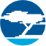# Traveo II Automotive Body Controller - FAQ – I2C - KBA232509Employee
Employee# 15. I2C

##### 15.1. How can the I2C configuration be evaluated based on actual Bus conditions?

Let us consider an example configuration of 400 KHz:

CLK_SCB = 10MHz or tCLK_SCB = 0.1 us.

AF_in = 0 , AF_out = 0, DF_in = 1.

The discussions are based on Glitch Filtering and  Oversampling and Bit Rate of 002-24401 Traveo™ II Automotive Body Controller High Family Architecture Technical Reference Manual (TRM)

Master:

Table 23-15 in Architecture TRM 002-24401 Rev. *C assumes worst case conditions on the I2C bus. The following equations can be used to determine the settings for your own system. This will involve measuring the rise and fall times on SCL and SDA lines in your system.

 tCLK_SCB(Min) = (tLOW + tF)/LOW_PHASE_OVS Equation 1

If clk_scb is any faster than this, tLOW of the I2C specification will be violated. Measure tF in your system.

 tCLK_SCB(Max) = (tVD – tRF – 100 ns)/3 (When analog filter is enabled and digital disabled) Equation 2 tCLK_SCB(Max) = (tVD – tRF)/4 (When analog filter is disabled and digital filter is enabled) Equation 3

Where, tRF is the maximum of either the rise or fall time.

If clk_scb is slower than this frequency, tVD will be violated.

Measure and evaluate your actual bus conditions to find the compatible configurations. To understand the compatibility, use Equation 1 to Equation 3.

Now, consider the timing parameters mentioned in the datasheet 002-21617 Rev. *G. For 400 KHz, see Table 25-10. Serial Communication Block (SCB) Specifications “I2C Interface-Fast-mode” in the datasheet. You can also find timing specifications in Figure 25-8. I2C Timing Diagrams in the datasheet 002-21617 Rev. *G.

Follow this approach for the timing parameters to be used in the equations:

• tF, tR – Measure in the bus.
• tLOW – Use theoretical value.
• tVD – Use spec value. tVD = tVD;DAT_F = tVD;ACK_F.

Based on section 6.1.3 Bit Rate Setting in 002-25401 Rev.*B:

• LOW_PHASE_OVS + HIGH_PHASE_OVS + 2 = 25
• LOW_PHASE_OVS + HIGH_PHASE_OVS = 23

Considering Table 23-15 in 002-24401 Rev. *C:

• LOW_PHASE_OVS range is [13,15]
• HIGH_PHASE_OVS range is [7,15]

Possible combinations are:

• LOW_PHASE_OVS 13, HIGH_PHASE_OVS 10
• LOW_PHASE_OVS 14, HIGH_PHASE_OVS 9
• LOW_PHASE_OVS 15, HIGH_PHASE_OVS 8

Consider an example, (LOW_PHASE_OVS, HIGH_PHASE_OVS) (15, 😎 and (13,10)

Assume tF = 100 ns, tR = 100 ns, tVD = 900ns, tLOW = 1250 ns.

Equation 1 and Equation 3 are the relevant equations, and are reiterated here:

 tCLK_SCB(Min) = (tLOW + tF)/LOW_PHASE_OVS Equation 1

 tCLK_SCB(Max) = (tVD – tRF)/4 (When analog filter is disabled and digital filter is enabled) Equation 3

1. Setting1: LOW_PHASE_OVS 15, HIGH_PHASE_OVS 8

tLOW = 1250 ns, tF = 100 ns, LOW_PHASE_OVS =15, tVD = 900 ns, tRF = 100 ns

tCLK_SCB (Min) = (1250 + 100)/15 = 90 ns = 0.09 us

tCLK_SCB (Max) = (900 – 100)/4 = 200 ns = 0.2 us

tCLK_SCB range is [0.09, 0.2]

Configured clock of tCLK_SCB 0.1 us falls within this range and there is no violation.

1. Setting2: LOW_PHASE_OVS 13, HIGH_PHASE_OVS 10

tLOW = 1250 ns, tF = 100 ns, LOW_PHASE_OVS =13, tVD = 900 ns, tRF = 100 ns

tCLK_SCB(Min) = (1250 + 100)/13 = 90 ns = 0.104 us

tCLK_SCB(Max) = (900 – 100)/4 = 200 ns = 0.2 us

tCLK_SCB range is [0.104, 0.2]

Configured clock of tCLK_SCB 0.1 us falls outside this range and there is violation.

Slave:

 tCLK_SCB(Max) = (tVD – tRF – 100 ns) / 3 (When analog filter is enabled and digital disabled) Equation 4

 tCLK_SCB(Max) = (tVD – tRF) / 4 (When analog filter is enabled and digital disabled) Equation 5

Where,

tRF is the maximum of either the rise or fall time. If clk_scb is slower than this frequency, tVD will be violated.

The minimum period of clk_scb is determined by one of the following equations:

 tCLK_SCB(MIN) = (tSU;DAT(min) + tRF)/16 (When analog filter is enabled and digital disabled) Equation 6

 tCLK_SCB(Min) = (0.6 * tF – 50 ns)/2 (When analog filter is enabled and digital disabled) Equation 7

 tCLK_SCB(Min) = (0.6 * tF)/3 (When analog filter is disabled and digital enabled) Equation 8

The result that yields the largest period from the above two sets of equations should be used to set the minimum period of clk_scb.

Equation 5 and Equation 6/Equation 8 are the relevant equations, and are reiterated here:

 tCLK_SCB(Max) = (tVD – tRF)/4 (When analog filter is enabled and digital disabled) Equation 5

 tCLK_SCB(MIN) = (tSU;DAT(min) + tRF)/16 (When analog filter is enabled and digital disabled) Equation 6

 tCLK_SCB(Min) = (0.6 * tF)/3 (When analog filter is disabled and digital enabled) Equation 8

1. Setting1: tRF = 100ns, tF = 100ns.

tF = 100ns, tVD = 900 ns, tRF = 100ns, tSU;DAT(min) = 100ns

tCLK_SCB(Max) = (900 – 100) /4 = 200 ns = 0.2 us

tCLK_SCB(Min)1 = (100 + 100)/ 16 = 12.5 ns = 0.0125 us

tCLK_SCB(Min)2 = (0.6 * 100)/ 3 = 20 ns = 0.02 us

tCLK_SCB(Min) = Max (tCLK_SCB(Min)1, tCLK_SCB(Min)2) = 0.02 us

tCLK_SCB range is [0.02, 0.2]

Configured clock of tCLK_SCB 0.1 us falls within this range and there is no violation.

1. Setting2: tRF = 300ns, tF = 300ns.

tF = 300ns, tVD = 900 ns, tRF = 300ns, tSU;DAT(min) = 100 ns

tCLK_SCB(Max) = (900 – 300) /4 = 150 ns = 0.15 us

tCLK_SCB(Min)1 = (100 + 300)/ 16 = 25 ns = 0.025 us

tCLK_SCB(Min)2 = (0.6 * 300)/ 3 = 60 ns = 0.06 us

tCLK_SCB(Min) = Max (tCLK_SCB(Min)1, tCLK_SCB(Min)2) = 0.06 us

tCLK_SCB range is [0.06, 0.15].

Configured clock of tCLK_SCB 0.1 us falls within this range and there is no violation.

538 Views